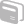Activities

•• Subject Area

• Maths: Number: Number
• Maths: Algebra: Algebra
• Maths: Multiple Representations: Multiple Representations

• Author11-14
14-16

2 Hours

• TI-Nspire™

TI-Nspire™

• Report an Issue

Trial and Improvement

Activity Overview

This activity allows learners to investigate the solution to an equation by trial and improvement. Learners are led through multiple representations of the approximate solution of a quadratic equation using the spreadsheet and graphing applications. The form of the quadratic is introduced using the data and statistics graphing facility.

Before the Activity

Some knowledge of substituting numbers in formulas and different forms of quadratic equation is required.

During the Activity

Objective: to investigate the solutions to a quadratic equation numerically and graphically.

After the Activity

This could lead into further work on the algebraic solution of quadratic equations.